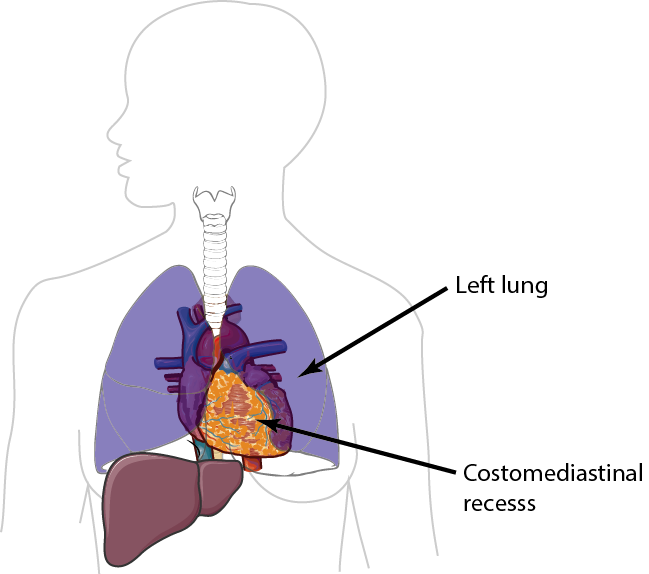Contents

# Contributing to MacDown

MacDown is open source and is a volunteer effort. This means that it depends on people to give some of their free time to improve it and make it even better.

If you are reading this, then you are probably curious or want to contribute in some way. Read on to see how you can do so.

## Getting the Source Code

MacDown is hosted on GitHub:

https://github.com/MacDownApp/macdown

Here you can get the source code, read through the issues and start contributing.

## But, I am not a Coder

Contribution is not limited to software developers, since there are other ways you can help. For example, contributing towards documentation, translation and support. Join the room on Gitter to see how you can help (see below).

If you want to help translate, then you can look at our project page on Transifex and see whether to add a new languages or complete the work of an existing language.

## Joining the Conversation

If you are new the project, then a good place to start is Gitter:

https://gitter.im/MacDownApp/macdown

Join the room, introduce yourself and find out how you can help out.

MacDown is released under the terms of MIT License. For more details take a look at the README.

## Testing

This is a test. $H_{2}O$. 2<sup>8</sup>\begin{equation} \tag{2} n = {\frac{2 \times (Z_{(1-\alpha/2)} – Z_{(1-\beta)})^2}{\delta^2}} \end{equation}

$$\Delta = \frac{\delta}{\sigma}$$

$[E=mc^2]$ $$(E=mc^2)$$

Syntax Description
$i\hbar\frac{\partial}{\partial t}\left|\Psi(t)\right>=H\left|\Psi(t)\right>$